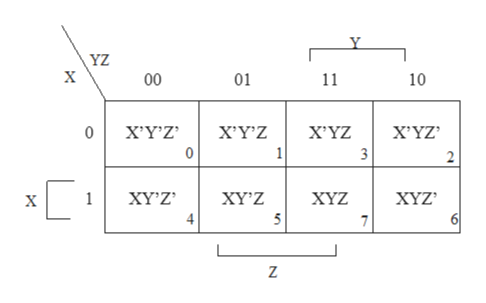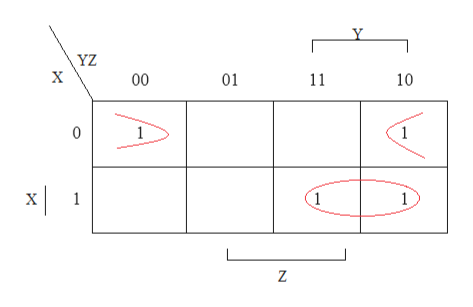# Simplify the following Boolean function , using three- variable maps:a) F(x,y,z) = Σ (0,2,6,7)b) F(A,B,C ) = Σ (0,2,3,4,6)c) F(a,b,c) = Σ (0,1,2,3,7)d) F(x,y,z) = Σ (3,5,6,7)

Question
55 views

Simplify the following Boolean function , using three- variable maps:

a) F(x,y,z) = Σ (0,2,6,7)

b) F(A,B,C ) = Σ (0,2,3,4,6)

c) F(a,b,c) = Σ (0,1,2,3,7)

d) F(x,y,z) = Σ (3,5,6,7)

check_circle

Step 1

Note: Since we only answer up to 3 sub-parts, we’ll answer the first 3. Please resubmit the question and specify the other subparts (up to 3) you’d like to answer.

Simplify the given Boolean function, using three- variable maps.

The boolean expression needs to simplify in order to reduce its complexity. The highest possible level of complexity is accomplished by the map.

The three-variable map ishelp_outlineImage TranscriptioncloseY YZ х 00 01 11 10 X'YZ Х'ҮZ 0 X'Y'Z X'YZ 0 1 2 XYZ 6 XҮ'Z 1 ΧYΖ ХYZ х 4 7 fullscreen
Step 2

a) F(x,y,z) = Σ (0,2,6,7)

Consider the minterms, 0, 2, 6, 7. The map for the minterms ishelp_outlineImage TranscriptioncloseY YZ х 00 01 11 10 0 1 1 1 1 х 1 fullscreen
Step 3

The first pattern is X’Y’Z’ and X’YZ’. The simple equation for this pattern is X’Z’.

The second pattern is XYZ and XYZ’. The simple equation for this pattern is XY.

Hen...

### Want to see the full answer?

See Solution

#### Want to see this answer and more?

Solutions are written by subject experts who are available 24/7. Questions are typically answered within 1 hour.*

See Solution
*Response times may vary by subject and question.
Tagged in

### Boolean Logic